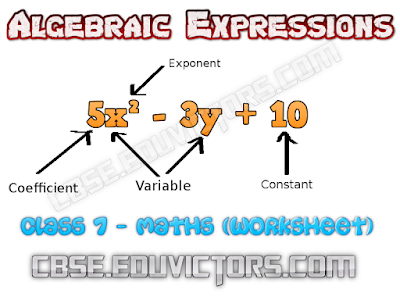## Algebraic Expressions

Class 7 - Mathematics

Worksheet

Fill in the blanks
Q1: An algebraic expression containing three terms is called a __________.

Q2: Factors of -5x²y²z are : _____________________________________.

Q3: Sum or difference of two like terms is a ______________.

Q4: -5a²b and -5b²a are ________________ terms.

Q5: In the expression 2πr, the algebraic variable is ___________ and 2π is ________.

Q6: Number of terms in a monomial is ________.

Q7: The speed of car is 55 km/h. The distance covered in y hours is ___________.

Q8: On adding a monomial __________ to -2x+4y²+z, the resulting expression becomes a binomial.

Q9: If Rohit has 5xy toffees and Shantanu has 20yx toffees, then Shantanu has ___________ more toffees.

Q10: Is (3a-b+3)-(a+b) is a binomial? (True/False)

Q11: The side length of the top of square table is 'a'. The expression for perimeter is ________.

Q12: Coefficient of x in -9xy²z is _________

Q13: The sum of 3x and 4y is 8. Express it in algebraic form.

Q14: 3x subtracted from 89 gives 44. Express it in algebraic form.

Q15: If 23 is a constant and x is a variable, 23 + x is constant. (True or False)

1: trinomial
2: 5 × x × x × y × y × z
3: like term
4: unlike terms
5: r, constant
6: one
7: 55y km
8: +2x or -4y² or -z
9: 15xy more toffees
10: False.
(3a-b+3)-(a + b)= 3a-b+3-a-b
= 3a-a-b-b + 3 = 2a-2b+ 3
The expression has three terms, it is a trinomial.

11: 4a
12: -9y²z
13: 3x + 4y = 8
14: 89 – 3x = 44
15: False. Sum of a constant and a variable is also variable.